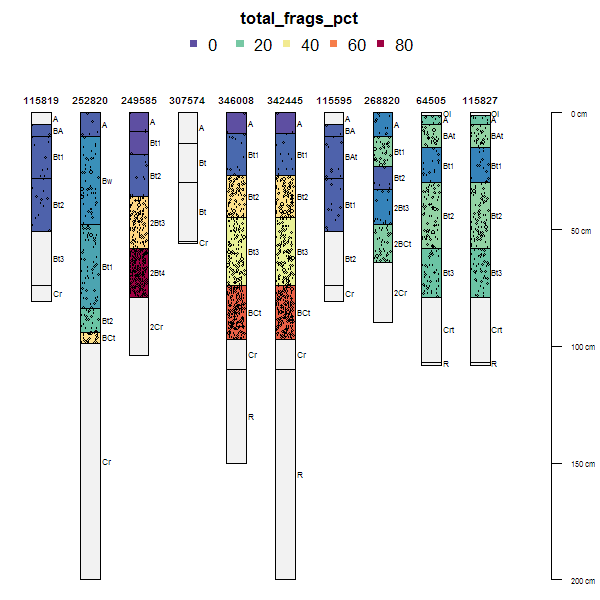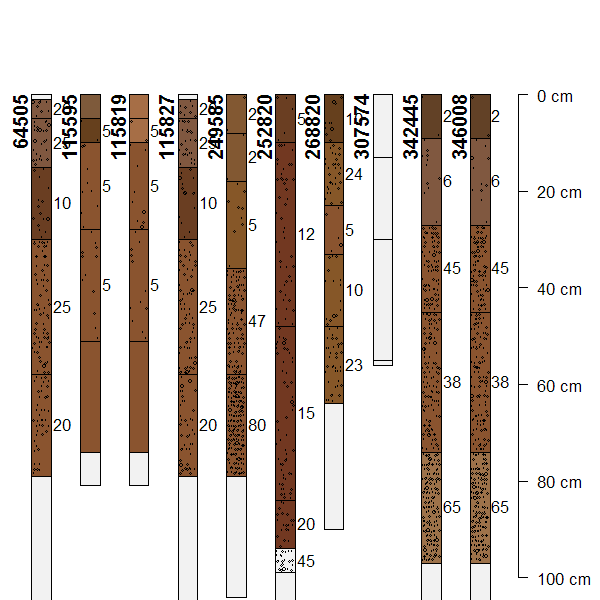addVolumeFraction {aqp} R Documentation

## Symbolize Volume Fraction on a Soil Profile Collection Plot

### Description

Symbolize volume fraction on an existing soil profile collection plot.

### Usage

```addVolumeFraction(x, colname, res = 10, cex.min = 0.1,
cex.max = 0.5, pch = 1, col = "black")
```

### Arguments

 `x` a `SoilProfileCollection` object `colname` character vector of length 1, naming the column containing volume fraction data (horizon-level attribute) `res` integer, resolution of the grid used to symbolize volume fraction `cex.min` minimum symbol size `cex.max` maximum symbol size `pch` plotting character `col` color of the symbol

### Details

This function can only be called after plotting a `SoilProfileCollection` object.

### Note

Details associated with a call to plot.SoilProfileCollection are automatically accounted for within this function: e.g. `plot.order`, `width`, etc.

### Author(s)

D.E. Beaudette

`plotSPC`

### Examples

``````# sample data
data(loafercreek, package='soilDB')

# subset first 10 profiles
s <- loafercreek[1:10, ]

# replace 0% frags with NA
s\$total_frags_pct[which(s\$total_frags_pct == 0)] <- NA

# plot in random order, note that annotations follow
par(mar=c(0, 0, 3, 0))
plot(s, color='total_frags_pct', plot.order=sample(1:length(s)))``````par(mar=c(0, 0, 0, 0))